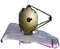# NIRSpec Predicted PerformanceNIRSpec sensitivity estimates may be obtained using the JWST Exposure Time Calculator (ETC). Methods on how to do this and and some best-estimates of limiting performance are available for users.

Information presented in the NIRSpec predicted performance articles describes the best knowledge of performance based on instrument component and flight model tests carried out on the ground. The scientific performance of all of JWST's instruments will be measured during the commissioning period immediately after launch.  The best estimates of the performance of all of JWST's instruments are incorporated into the JWST Exposure Time Calculators (ETC). The information provided on predicted sensitivity performance within these pages is only for general guidance. Measured on-sky performance values will be incorporated into the ETCs and provided here as they become available.

Related NIRSpec predicted performance information can be found in these articles:

The calculation method for sensitivity, transmission of the combined optical element and the efficiency of the detectors is discussed below.

# From surface brightness to quantized units

The information shown here is meant as a guideline for the NIRSpec calculations in the linked subpages: NIRSpec Sensitivity and Bright Source Limits. The JWST Exposure Time Calculators (ETC) should be used to derive the best estimate of signal-to-noise and saturation limits for science sources of interest.

The the flux rate at the NIRSpec entrance aperture, $\begin{array}{l}S_\lambda^{ideal}\end{array}$, is derived from the surface brightness of the astronomical source of interest $\begin{array}{l}(I_\lambda)\end{array}$, the telescope collecting area (A) in cm2 and the dimensionless combined transfer efficiency of the OTE (primary, secondary, tertiary, and fine steering mirrors) $\begin{array}{l}(\eta_{OTE}(\lambda))\end{array}$.

 (5)

where $\begin{array}{l}S_\lambda^{ideal}\end{array}$  is in units of  photons/s/μm/pixel.

After the OTE, the flux is transferred through a specific instrument optics system.

Instrument optics systems are a crucial telescope component that can be described by one-dimensional (wavelength-dependent) efficiency curves (unity is perfect transmission). Each instrument/mode combination can have efficiency contributions from internal reflections and optical stops, filters, and dispersers. The actual focal plane flux rate is then:

 (6)

in units of photons/s/μm/pixel.

Faint sensitivity limits for a specific benchmark calculation case are described in the NIRSpec Sensitivity article. The signal to noise is derived from the number of incident photons measured by the end to end instrument, including the detector. This optical system transmission, $\begin{array}{l}S_\lambda\end{array}$, follows from the above equation via a detector quantum efficiency function (QE; see the upper curve in Figure 1). The QE curve assumes a quantum yield (QY) of unity at all wavelengths (i.e. the efficiency of a detector at converting an incident photon to a measured electron is 1.0).

The optical transmission function is:

 (7)

in units of e/s/μm/pixel. The optical transmission is presented in Figure 2 for the NIRSpec grating-filter combinations using both the MOS internal optics throughput and the IFU internal optics throughput.  Note that fixed slit (FS) spectroscopy shares the same optics as MOS.

Investigation of bright saturation limits for the NIRSpec detectors is described in the NIRSpec Bright Limits article. The calculations presented in the bright limit case measure the number of electrons that accumulate in the detector, based on the incident photons. In this case, the photon conversion efficiency (PCE) is used to present overall system efficiency of detecting electrons and filling the detector well. Calculation of the PCE uses the detector relative quantum efficiency (RQE), which is the QE multiplied by the quantum yield of the detector.  At wavelengths shortward of 1.4 μm, a single incident photon can result in more than one electron measured on the detector. This results in a RQE greater than 1.0, as presented in the lower panel of Figure 1.

The PCE is:

 (8)

in units of e/s/μm/pixel. The PCE is shown in Figure 3 for the MOS and the IFU internal optics throughputs.The upper plot presents the NIRSpec quantum efficiency (QE) as a function of wavelength expressed in units of e–/photon. Maximum efficiency is unity. The QE curve assumes a quantum yield (QY; efficiency of a detector at converting an incident photon to a measured electron) of unity at all wavelengths. The lower plot presents the relative quantum efficiency of the detector, RQE. This is the product of the QE and the actual quantum yield, which is not always unity. At wavelengths shortward of 1.4 μm the RQE is greater than 1.0 because an incident photon can result in more than one measured electron on the detector.The NIRSpec transmission function for a set of grating-filter combinations for the MOS observing mode (upper plot) and the IFU observing mode (lower plot). The vertical axes shows the product of the efficiency of the filter, the efficiency of the grating, the efficiency of the MOS or IFU configuration, and the detector quantum efficiency (QE) as a function of wavelength expressed in μm.    Fixed slit (FS) spectroscopy with NIRSpec uses the same optics as MOS.NIRSpec photon conversion efficiency (PCE) for a set of grating-filter combinations for the MOS observing mode (upper plot) and the IFU observing mode (lower plot). The vertical axes shows the product of the efficiency of the filter, the efficiency of the grating, the efficiency of the MOS or IFU configuration, and the detector relative quantum efficiency (RQE; including quantum yield) as a function of wavelength expressed in μm. It is evident from comparison with the previous figure that the quantum yield has the biggest effect at short wavelengths (the G140 band).  FS spectroscopy with NIRSpec uses the same optics as MOS.

# References

Pontoppidan, K. 2016, Proc of SPIE, 9910, 16
Pandeia: a multi-mission exposure time calculator for JWST and WFIRST

Published 23 Jan 2018